Neural Network Control of Asymmetrical Multilevel Converters

Rachid TALEB1*, Abdelkader MEROUFEL2, Patrice WIRA3

1Electrical Engineering Department, Hassiba Ben Bouali University BP 151 Hay Es-Salam Chlef, Algeria.

2Intelligent Control and Electrical Power Systems Laboratory (ICEPS) Djillali Liabes University, BP 89 Sidi Bel-Abbes, Algeria.

3Laboratoire Modélisation, Intelligence, Processus et Systèmes (MIPS) Université de Haute Alsace, 68093 Mulhouse, France.

(* Corresponding author)

Abstract

This paper proposes a neural implementation of a harmonic elimination strategy (HES) to control a Uniform Step Asymmetrical Multilevel Inverter (USAMI). The mapping between the modulation rate and the required switching angles is learned and approximated with a Multi-Layer Perceptron (MLP) neural network. After learning, appropriate switching angles can be determined with the neural network leading to a low-computational-cost neural controller which is well suited for real-time applications. This technique can be applied to multilevel inverters with any number of levels. As an example, a nine-level inverter and an eleven-level inverter are considered and the optimum switching angles are calculated on-line. Comparisons to the well-known sinusoidal pulse-width modulation (SPWM) have been carried out in order to evaluate the performance of the proposed approach. Simulation results demonstrate the technical advantages of the proposed neural implementation over the conventional method (SPWM) in eliminating harmonics while controlling a nine-level and eleven-level USAMI. This neural approach is applied for the supply of an asynchronous machine and results show that it ensures a highest quality torque by efficiently canceling the harmonics generated by the inverters.

Keywords

Uniform step asymmetrical multilevel inverter; Harmonics elimination strategy; Artificial neural networks; Multi-Layer Perceptron; Sinusoidal pulse-width modulation.

Introduction

Multilevel inverters synthesize a desired ac-voltage from several levels of dc-voltages. For this reason, multilevel inverters are of a great focus for being inserted either in series or in parallel in modern power grids with distributed energy resources  or in power quality controllers . Three-level inverters are conventional apparatus but other topologies have been attempted this last decade for different kinds of applications . Among them, neutral point clamped inverters, flying capacitors inverters also called imbricated cells, and series connected cells inverters also called cascaded inverters [4, 5].

Three-phase multilevel converters based on series connected single phase inverters (partial cells) in each phase are studied in this work. A multilevel converter with k partial inverters connected in serial is presented by Fig. 1. In this configuration, each cell of rank j = 1, ...k is supplied by a dc-voltage source udj. It has been shown that feeding partial cells with unequal dc-voltages (asymmetric feeding) increases the number of levels of the generated output voltage without any supplemental complexity to the existing topology . These inverters are referred to as "Asymmetrical Multilevel Inverters" or AMI.

Some applications such as active power filtering need inverters with high performances . These performances are obtained if there are still any harmonics at the output voltages and currents. Different Pulse-Width Modulation (PWM) control-techniques have been proposed in order to reduce the residual harmonics at the output and to increase the performances of the inverters [8, 9]. The most popular one is probably the sinusoidal PWM technique  which shifts the harmonics to high frequencies by using high-frequency carriers. However, electronic devices and components have limited switching-frequencies. High-frequency carriers are therefore limited by this constraint. An alternative solution consists in adapting the principle of the Harmonics Elimination Strategy (HES) to AMIs. The HES allows canceling the critical harmonic distortions and therefore controlling the fundamental component of the signal by using electronic devices with low switching frequencies. This is particularly useful when feeding asynchronous machines. Clear control signals increase the performances of the motor tasks .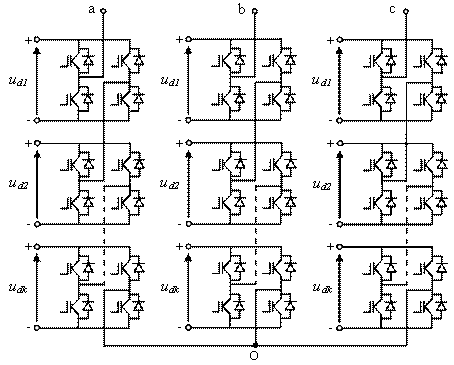Figure 1. Three-phase structure of a multilevel converter with k partial monophased inverters series connected per phase

The principle of the HES relies on the resolution of a system of non linear equations in order to elaborate the switching angle control signals for the electronic devices . Practically, the implementation of this method requires memorizing all the firing angles which is complex and needs considerable computational costs. Mathematical solutions with limited computational costs are therefore preferably used for real-time applications. The approach can be achieved with Artificial Neural Networks (ANNs) which are known as parsimonious universal approximators. Their learning from examples leads to robust generalization capabilities .

A HES based on ANNs to control high-levels Uniform Step Asymmetrical Multilevel Inverters (USAMIs) is proposed in this paper. The developments are provided for a 11-level USAMI but the approach is very general and is based on a standard Multi-Layer Perceptron (MLP) . The neural network is employed for approximating the relationship between the modulation rate and the inverters switching angles. The performance of this neural approach is evaluated with inverters of 9 and 11 levels. The performances are compared to the ones obtained with the conventional sinusoidal PWM technique. The proposed neural strategy is also evaluated when the inverter supplies an asynchronous machine. In this application, it is important that the implemented controller computes appropriate switching angles for the inverter in order to minimize the harmonics absorbed by the asynchronous machine. Performances where successfully achieved, the neural controller demonstrates a satisfying behaviour and a good robustness.

The structure of the paper is as follows. USAMIs are described and modeled in Section 2. Section 3 briefly introduces the well-known sinusoidal PWM and brings out the original HES based on a MLP. Section 4 evaluates the proposed neural strategy in computing optimal angles of 9 and 11-level inverters used to supply an asynchronous machine. Results show that the neural method cancels the harmonic distortions and supplies the machine with a well-formed sinusoidal voltage waveform. In Section 5, we conclude with final remarks.

Uniform Step Asymmetrical Multilevel Inverters

Asymmetric multi-level inverters use different intermediate-circuit capacitor voltages in various parts of the inverter. By addition and subtraction of these voltages, more different output-voltage levels can be generated with the same number of components compared to symmetric multi-level inverters. Multilevel inverters generate at the ac-terminal several voltage levels as close as possible to the input signal. The output voltage step is defined by the difference between two consecutive voltages. A multilevel converter has a uniform or regular voltage step, if the steps ∆u between all voltage levels are equal. In this case the step is equal to the smallest dc-voltage, ud1 . This voltage can be expressed by: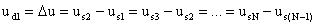(1)

with N voltage levels. If this is not the case, the converter is called a non uniform step AMI or irregular AMI. An USAMI is based on dc-voltage sources to supply the partial cells (inverters) composing its topology which respects the following conditions :(2)

where k represents the number of partial cells per phase and j = 1, ...k. The number of levels of the output voltage can be deduced from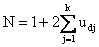(3)

This relationship fundamentally modifies the number of levels generated by the multilevel topology. Indeed, the value of N depends on the number of cells per phase and the corresponding supplying dc-voltages.

The expression given by (3) accepts different solutions. With k = 3 for example, there are two possible combinations of supply voltages for the partial inverters in order to generate a 11-level global output, i.e., (ud1, ud2, ud3) Î {(1, 1, 3); (1, 2, 2)}, and there are three possible combinations to generate a 15-level global output, i.e., (ud1, ud2, ud3) Î {(1, 1, 5); (1, 2, 4); (1, 3, 3)}. Fig. 2 shows the possible output voltages of the three partial cells of the 11-level inverter with k = 3. The dc-voltages of the three cells are ud1 = 1p.u., ud2 = 2p.u. and ud3 = 2p.u. The output voltages of each partial inverter are noted up1, up2 and up3 and can take three different values: up1 Î {-1, 0, 1}, up2 Î {-2, 0, 2} and up3 Î {-2, 0, 2}. The result is a generated output voltage with 11 levels: us Î {-5, -4, -3, -2, -1, 0, 1, 2, 3, 4, 5}. Some levels of the output voltage can be generated by different commutation sequences. For example, there are three possible commutation sequences resulting with us = 3p.u. These sequences are the followings: (up1, up2, up3) Î {(-1, 2, 2); (1, 0, 2); (1, 2, 0)}. One of these redundant combinations can be preferred by considering that the switching process of the inverter can be optimized . These different possibilities offered by the output voltage of the partial inverters, and the redundancies among them to deliver a same output voltage level, can be considered as degrees of freedom which can be exploited in order to optimize the use of an AMI.Figure 2. Possible output voltages of each partial inverter for generate N = 11 levels with k = 3 cells per phase (with ud1 = 1 p.u., ud2 = 2 p.u. and ud3 = 2p.u.)

Multilevel Inverters Control Strategies

Among several modulation strategies, the multi-carrier sub-harmonic PWM technique has been receiving an increasing attention for symmetrical multilevel converters . This modulation method can also be used to control asymmetrical multilevel power converters. Other kinds of modulation techniques can also be used in the case of AMIs .

This section briefly presents the sinusoidal PWM technique. We also propose HES based on ANNs. These control strategies will be compared by computer simulations. The objective is to elaborate optimized switching angles for a 11-level USAMI in order to supply an asynchronous machine.

Sinusoidal Pulse-Width Modulation (SPWM)

The Sinusoidal PWM (SPWM) is also known as the multi-carrier PWM because it relies on a comparison between a sinusoidal reference waveform and vertically shifted carrier waveforms. N -1 carrier is therefore required to generate N levels. The carriers are in continuous bands around the zero reference. They have the same amplitude Ac and the same frequency fc. The sine reference waveform has a frequency fr and an amplitude Ar. At each instant, the result of the comparison is 1 if the triangular carrier is greater than the reference signal and 0 otherwise. The output of the modulator is the sum of the different comparisons which represents the voltage level. The strategy is therefore characterized by the two following parameters , respectively called the modulation index and the modulation rate: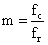(4)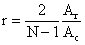(5)

We propose to develop a 11-level inverter composed of k = 3 partial inverters per phase with the following dc-voltage sources: ud1 = 1 p.u., ud2 = 2 p.u. and ud3 = 2 p.u. Without loss of generality, we used Ud1 = 70V and Ud2 = Ud3 = 140V. The frequency representation of output voltage Vab generated with the SPWM is represented by Fig. 3.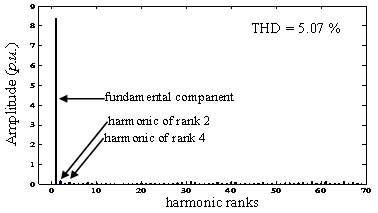Figure 3. Frequency content of the output voltage Vab with the sinusoidal PWM strategy  (with r = 0.965 and m = 30)

Neural Approach of Harmonics Elimination

The neural approach for cancelling the harmonics is based on the principle of the HES. The HES evaluates the generated voltage us at the output of the USAMI with the Fourier theory . This voltage is symmetric in a half and a quarter of a period. As a result, the even harmonic components are null. The Fourier series expansion for the us voltage is thus:(6)

where un represents the amplitude of the harmonic term of rank n, p = (N - 1)/2 is the number of switching over a quarter of a period, and qi are the switching angles (i = 1, 2...,p).

The p switching angles in (6) are calculated by fixing the amplitude of the fundamental term and by cancelling the p − 1 other harmonic terms. Practically, five switching angles (q1, q2 q3, q4, q5) are necessary for cancelling the four first harmonics terms (i.e., harmonics with a odd rank and non multiple of 3, therefore 5, 7, 11 and 13) in the case of a three phase 11-level USAMI composed of k = 3 partial inverters per phase supplied by the dc-voltages ud1 = 1p.u., ud2 = 2p.u. and ud3 = 2p.u. These switching angles can be determined by solving the following system of non linear equations:(7)

where r = u1/5ud1 is the modulation rate. The solution of (7) must also satisfy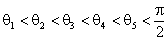(8)

and can be solved by applying the Newton-Raphson method. This method returns all the possible combinations of the switching angles for different values of r. The result is represented by Fig. 4 a) where one can see the presence of two possible solutions of angles for 0.65 ≤ r ≤ 0.74 and for 0.77 ≤ r ≤ 0.89. On the other side, the system does not accept any solution for r < 0.48 and 0.92 ≤ r ≤ 0.951. The system has a unique solution for all the other values of r.

In the case of two possible solutions for an angle qi, the criteria for selecting one of them can be the Total Harmonic Distortion (THD). The best angle values are therefore the ones leading to the lowest THD. The THD is a quantifiable expression for determining how much the signal has been distorted. The greater are the amplitudes of the harmonics, the greater are the distortions. The THD is defined by:(9)

The THD was calculated for all possible angles and Fig. 5 a) shows the THD according to the modulation rate and with switching angles leading to the lowest THD.

The control of an AMI with the HES in real-time applications requires memorizing the switching angles sequence that is necessary to allow the output to commutate naturally without distortion. A considerable computational memory space must therefore be involved for the implementation of this control law. This incompressible burden does generally not meet the constraints on the computing resources of real-time applications.

We propose an implementation of the HES with ANNs. ANNs have gained increasing popularity and have demonstrated superior results compared to alternative methods in many studies. Indeed, ANNs are able to map underlying relationship between input and output data without prior understanding of the process under investigation. This mapping is achieved by adjusting their internal parameters called weights from data. This process is called the learning or the training process. Their interest comes also from their generalization capabilities, i.e., their ability to deliver estimated responses to inputs that were not seen during training. Hence, the application of ANNs to complex relationships and processes makes them highly attractive for different types of modern problems [13, 17].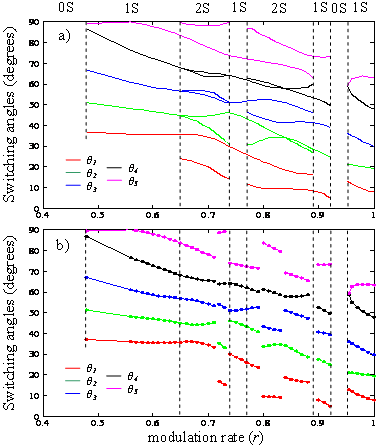Figure 4. Representation of the switching angles according to r, a) angles calculated with the Newton-Raphson method, b) angles estimated by the MLP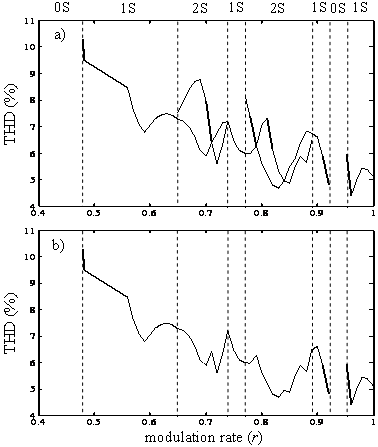Figure 5. Representation of the THD according to r, a) THD resulting from the Newton-Raphson method, b) THD resulting from learning with the MLP

We use a neural network to learn the switching angles previously provided by the Newton-Raphson method. The approach aims to replace the painful memorization of the angles in order to make its implementation realizable in a real-time application. The neural approach enjoys a capability of self-tuning to the plant properties and offers high quality of the resultant compensation. The main idea is to use output as source of information on the quality of the compensation through the resulting THD.

MLPs  are well suited for learning and approximating multidimensional and nonlinear relationships. Associated to the back propagation learning rule, they are known as universal approximations .

An MLP network is composed of a number of identical units called neurons organized in layers, with those on one layer connected to those on the next layer, except for the last layer or output layer. Indeed, MLPs architecture is structured into an input layer of neurons, one or more hidden layers and one output layer. Neurons belonging to adjacent layers are usually fully connected and the activation function of the neurons is generally sigmoid or linear. In fact, the various types and architectures are identified both by the different topologies adopted for the connections and by the choice of the activation function. A complete network for a 3 ´ 5 ´ 2 functional mapping is shown by Fig. 6 as an example.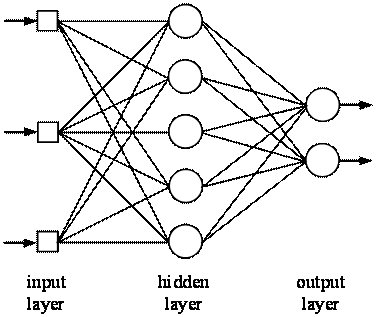Figure 6. A feedforward Multi-Layer Perceptron (MLP) using sigmoid-type neurons

(with 3 inputs, 5 and 2 neurons in the hidden and output layers respectively)

Some parameters of ANNs can not be determined from an analytical analysis of the process under investigation. This is the case of the number of hidden layers and the number of neurons belonging to them. Consequently, they have to be determined experimentally according to the precision which is desired for the estimation. The number of inputs and outputs depends from the considered process. In our application, the MLP has to map the underlying relationship between the modulation rate (input) and the p switching angles (output). The MLP is thus composed by one input neuron and p output neurons.

The MLP must be trained in order to adjust and to find the adequate weights. This is achieved by using probabilistic learning techniques and with data from the process under investigation. The training data consists of the inputs R and the corresponding desired output vectors S which are: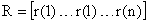(10)(11)

In the last two expressions, l = 1... n, where n is the number of examples. For a given input r(l), the MLP computes an estimated output vector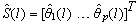that must be as close as possible to the ideal desired output S(l). The difference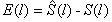constitutes the estimation error for example l that is used by the training algorithm to correct the weights of the neurons. This is repeated for the n samples composing the training data set until convergence is reached. The learning is achieved with the back propagation algorithm .

After the training process, the MLP is able to estimate the angles corresponding to an input r(l). In other words, the MLP has learned the functions qi = fi(r) with i = 1... 5. By approximating these functions, the MLP will be able to deliver the angles for the real-time control of the inverter.

Test Results

The effectiveness of the proposed neural method is evaluated by various simulation tests. In this section, the performance of neural HES is assessed for both, a 9 and a 11-level USAMI even if the previous developments were detailed for a 11-level USAMI. The error in estimating the angles with the neural network is examined in first. Its ability for providing the optimal angles to control the USAMI is then evaluated. The working of the neural HES is also carefully tested in feeding an open-loop asynchronous machine with a 9 or 11-level USAMI. These tests are detailed thereafter.

Learning performances

The MLP is applied for cancelling harmonics 5, 7, 11 and 13 at the output of the USAMI. The training set is elaborated from the Newton-Raphson method and the optimal angles are the ones resulting in the lowest THD when several solutions exist.

An MLP with one hidden layer is used with a training set of n = 43 examples. Several tests have been conducted for determining the number of neurons of the hidden layer. Results are provided by Table 1. These tests have been achieved because there are no generally acceptable theories, solutions being in the specific literature only for special cases. The determination of number of neurons in hidden layers is very important as it affects the training time and generalization property of neural networks.

Table 1. Approximation errors with various sizes of the MLP

 MLP architecture Required iterations Learning error 1 ´ 3 ´ 5 10000 3296 1 ´ 5 ´ 5 10000 2447 1 ´ 8 ´ 5 10000 1576 1 ´ 10 ´ 5 7326 0.829 1 ´ 12 ´ 5 4862 0.168 1 ´ 14 ´ 5 3971 7∙10-3 1 ´ 15 ´ 5 2320 5∙10-3 1 ´ 16 ´ 5 1934 1∙10-3

According to the results given by Table 1, a MLP with 16 neurons in the hidden layer has been adopted. The approximating error remains the same with 16 neurons as with a higher number of neurons in the hidden layer. This configuration has been chosen after different experiments, it represents the best compromise between computational costs and performances. The other parameters of the MLP are detailed in Table 2.

Table 2. Properties of the MLP

 MLP parameters values Network configuration 1 ´ 16 ´ 5 Transfer functions tansig, purelin Training technique Levenberg-Marquardt Learning rate 0.1 Momentum constant 0.9 Training goal 0.001 Training examples 43 Iterations 1934 Maximum iterations 10 000

The learning convergence of the 1´16´5-MLP is reached after 1934 iterations, and leads to angle errors less than 0.001 degrees. The outputs delivered by the MLP are therefore very close to the angles given by the Newton-Raphson method. The estimated angles are represented by Fig. 4b) and the resulting and optimized THD by Fig. 5b). The curves presented by these figures can be compared to the theoretical solutions represented by Fig. 4a) and Fig. 5a) respectively.

After learning, the MLP is also able to deliver the angles for inputs which were not present in the training set. Theses generalization capabilities are very interesting in our application, the neural controller is therefore always able to deliver the control signals for the inverter. For example, Fig. 7 shows the results obtained for an input which was not in the training set, r(l) = 0.965 which theoretically corresponds to q1 = 11.0242°, q2 = 20.7792°, q3 = 34.3378°, q4 = 53.4882° and q5 = 63.0578°. Fig. 8 shows the frequency content of Vab which can be compared to Fig. 3 obtained with the SPWM.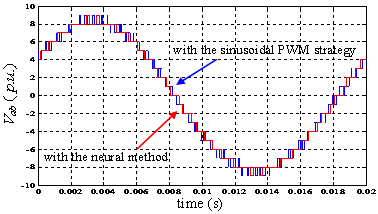Figure 7. Output voltage Vab of the 11-level USAMI controlled by the sinusoidal

PWM (with r = 0.965 and m = 30) and the neural HES (with r(l) = 0.965 and p = 5)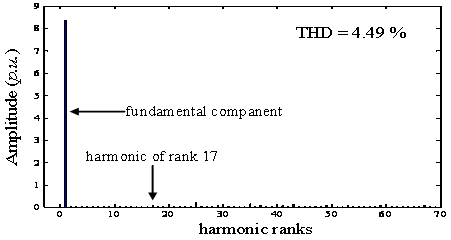Figure 8. Frequency content of Vab with the proposed neural HES

(with r(l) = 0.965 and p = 5)

Performance in feeding an asynchronous machine with a 11-level inverter

In this test, a 11-level USAMI is used to supply an asynchronous machine in order to evaluate the performance and the robustness of the proposed approach in computing optimized control signals, i.e., that are substantially free of harmonics. We used an asynchronous machine with the following data: Rs = 4.850W, Rr = 3.805W, Ls = Lr = 0.274H, Lm = 0.258H, P = 2, J = 0. 031 kg.m2, and viscous friction coeff. Kf = 0.00136 Nm.s.rad-1.

The neural HES is compared to the sinusoidal PWM strategy in controlling the 11-level USAMI. The objective is to use the proposed neural strategy in order to minimize the harmonics absorbed by the asynchronous machine.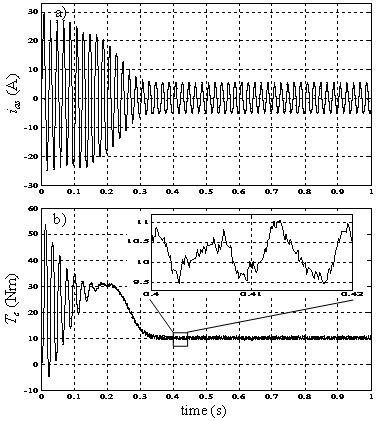Figure 9. Stator current (a) and electromagnetic torque (b) of the asynchronous

machine fed by a 11-level USAMI controlled by the sinusoidal PWM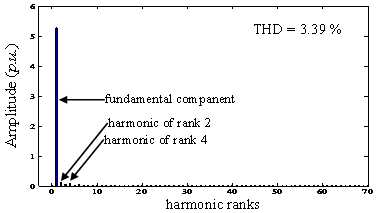Figure 10. Frequency content of the stator current ias of the asynchronous machine

fed by a 11-level USAMI controlled by the sinusoidal PWM

The results of the control based on the sinusoidal PWM are presented by Fig. 9 and Fig. 10. This first figure shows the stator current and the electromagnetic torque with significant fluctuations. The second figure shows the frequency content of the stator current.

Results by using neural approach with the MLP issued for the previous learning process are presented by Fig. 11 and Fig. 12. By comparing Fig. 10 to Fig. 12, it can be deduced that the neural HES efficiently cancels the harmonics of rank 5, 7, 11 and 13 from the output voltage Vab. Moreover, the amplitudes of the harmonic distortions are very small compared to the amplitude of the fundamental component.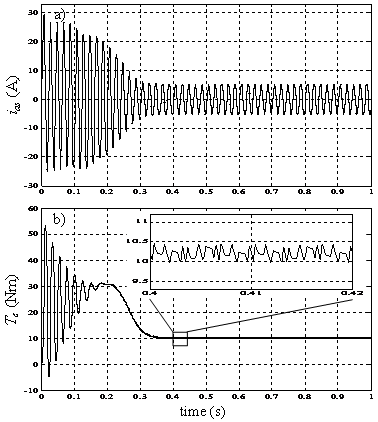Figure 11. Stator current (a) and electromagnetic torque (b) of the asynchronous

machine fed by a 11-level USAMI controlled by the proposed neural HES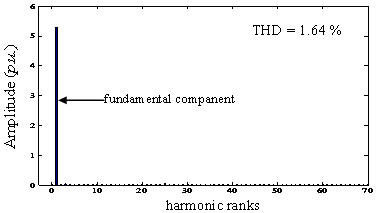Figure 12. Frequency content of the stator current ias of the asynchronous machine

fed by a 11-level USAMI controlled by the proposed neural HES

Performances obtained with both methods are summarized in Table 3. The THD measured on Vab and resulting from the neural approach of the HES is smaller than the one obtained with the sinusoidal PWM method. The THD measured on the stator current ias is reduced by a factor 2.06 with the neural HES compared to the sinusoidal PWM method. The control is thus optimized with the neural HES in order to avoid the asynchronous machine to absorb harmonics.

It can also be seen that the electromagnetic torque continuously oscillates at a frequency f with the sinusoidal PWM method (because of the harmonics of rank 2 and 4 which are present in the output voltage). The torque oscillates at 2f with the neural approach. The neural method also reduced the number of switching angles by a factor 3 compared to the sinusoidal PWM method which is highly appreciated for the electronic devices.

Table 3. Control performance a 11-level inverter

 Control method Vab THD (%) ias THD (%) f Te (Hz) ∆Te (Nm) Nb of qi 11-level sin PWM 5.07 3.39 f 1.60 2m = 60 11-level neural HES 4.49 1.64 2f 0.45 4p = 20

Performance in feeding an asynchronous machine with a 9-level inverter

The same asynchronous machine has been feed with a 9-level USAMI controlled with the neural HES. This allows comparing the performances of the neural HES in controlling a 9-level USAMI and a 11-level USAMI.

Table 4. Control performance a 9-level inverter

 Control method Vab THD (%) ias THD (%) f Te (Hz) ∆Te (Nm) Nb of qi 9-level sin PWM 9.19 5.05 f 1.77 2m = 48 9-level neural HES 7.66 1.92 2f 1.31 4p = 16

Control results with the 9-level USAMI are synthesized in Table 4. They can be compared the ones previously presented with the 11-level USAMI (in Table 3). As can be expected, the 11-level USAMI is able to synthesize voltage waveforms with a reduced harmonic content. Indeed, with the neural HES, the THD of the output voltage Vab is 4.49 % with the 11-level USAMI and 7.66 % 9-level USAMI. The harmonic distortions are also cancelled in the stator current ias with the 11-level USAMI when compared to the 9-level USAMI. Furthermore, the electromagnetic torque oscillations DTe are considerably been reduced with the 11-level USAMI and the proposed neural HES.

Conclusions

The performance of motors feed by inverters are closely related to the strategy used to control its supplying inverter. Indeed, the control currents of the motor can be disturbed by harmonics introduced by the inverter and Harmonics Elimination Strategies (HES) are generally used. We propose a low-cost neural implementation of HES to control a 9-level and 11-level USAMI. The approach is based on the learning and approximating of the relationship between the modulation rate and the switching angles with a Multi-Layer Perceptron. The resulting neural implementation of the HES uses very few computational costs. It is particularly well suited for real-time motor control tasks. The proposed neural approach is compared to the SPWM strategy. Simulation results are given to show the high performance and technical advantages of the neural implementation of the HES for the control of a 9-level and 11-level USAMI. The proposed neural method efficiently cancels the current harmonic distortions in supplying an asynchronous machine. As a result, the torque undulations and the switching losses are significantly reduced.

References

1.            Selvaraj J., Rahim N. A., Multilevel Inverter For Grid-Connected PV System Employing Digital PI Controller, IEEE Transactions on Industrial Electronics, 2009, 56(1), p. 149-158.

2.            Zhou G., Wu B., Xu D., Direct Power Control of a Multilevel Inverter Based Active Power Filter, Electric Power Systems Research, 2007, 77(3-4), p. 284-294.

3.            Rodriguez J., Lai J.S., Peng F.Z., Multilevel Inverters: A Survey of Topologies, Controls, and Applications, IEEE Transactions on Industrial Electronics, 2002, 49(4), p. 724-738.

4.            Meynard T. A., Fadel M., Aouda N., Modeling of Multilevel Converters, IEEE Transactions on Industrial Electronics, 1997, 44(3), p. 356-364.

5.            Manjrekar M. D., Topologies, Analysis, Controls and Generalization in H-Bridge Multilevel Power Conversion, Ph.D. dissertation, University of Wisconsin, Madison, 1999.

6.            Mariethoz S., Etude formelle pour la synthèse de convertisseurs multiniveaux asymétriques: topologies, modulation et commande, Ph.D. dissertation (in french), EPFL, Lausanne, Switzerland, 2005.

7.            Abdeslam D. O., Wira P., Merckl J., Flieller D., Chapuis Y. A., A unified artificial neural network architecture for active power filters, IEEE Transactions on Industrial Electronics, 2007, 54(1), p. 61-76.

8.            McGrath B. P., Holmes D. G., Multicarrier PWM Strategies for Multilevel Inverters, IEEE Transactions on Industrial Electronics, 2002, 49(4), p. 858-867.

9.            Cochowski A., Nieznznski J., Self-Tuning Dead-Time Compensation Method for Voltage-Source Inverters, IEEE Power Electronics Letters, 2005, 3(2), p. 72-75.

10.        Song-Manguelle J., Mariethoz S., Veenstra M., Rufer A., A Generalized Design Principle of a Uniform Step Asymmetrical Multilevel Converter for High Power Conversion, European Conference on Power Electronics and Applications, Graz, Austria, 2001.

11.        Saied S. A., Abbaszadeh K., Cogging Torque Reduction in BLDC Motors and PMSM Using Dual Structures, International Review on Modelling and Simulations, 2009, 2(1).

12.        Chiasson J.N., Tolbert L.M., McKenzie K.J., Du Z., A unified approach to solving the harmonic elimination equations in multilevel converters, IEEE Transactions on Power Electronics, 2004, 19(2), p. 478-490.

13.        Haykin S., Neural Networks: A comprehensive Foundation, 2nd ed. Prentice Hall, 1999.

14.        Bishop C.M., Neural Networks for Pattern Recognition, Clarendon Press, 1995.

15.        Song-Manguelle J., Convertisseurs multiniveaux asymétriques alimentés par transformateurs multi-secondaires basse-fréquence: réactions au réseau d’alimentation,  Ph.D. dissertation (in french), EPFL, Lausanne, Switzerland, 2004.

16.        Dahidah M. S. A., Agelidis V. G., Selective Harmonic Elimination PWM Control for Cascaded Multilevel Voltage Source Converters: A Generalized Formula, IEEE Transactions on Power Electronics, 2008, 23(4), p. 1620-1630.

17.        Khomfoi S., Tolbert L. M., Fault Diagnostic System for a Multilevel Inverter Using a Neural Network, IEEE Transactions on Power Electronics, 2007, 22(3), p. 1062-1069.